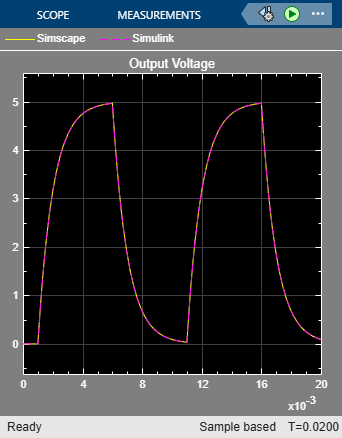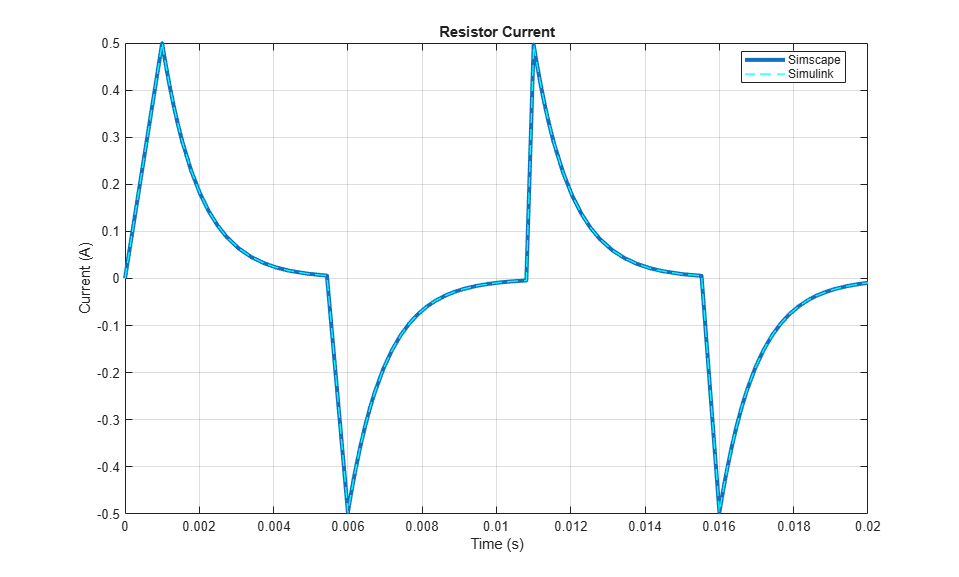# RC Circuit in Simulink and Simscape

This example shows two models of an RC circuit, one using Simulink® input/output blocks and one using Simscape™ physical networks.

The Simulink uses signal connections, which define how data flows from one block to another. The Simscape model uses physical connections, which permit a bidirectional flow of energy between components. Physical connections make it possible to add further stages to the RC circuit simply by using copy and paste. Input/output connections require rederiving and reimplementing the circuit equations.

The circuit is driven by a voltage square wave. Resistance and capacitance values are defined using MATLAB® variables.

### Model### Simulation Results from Scopes### Simulation Results from Simscape Logging